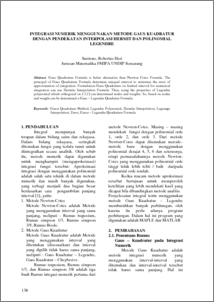# INTEGRASI NUMERIK MENGGUNAKAN METODE GAUS KUADRATUR DENGAN PENDEKATAN INTERPOLASI HERMIT DAN POLINOMIAL LEGENDRE

Sutrisno, Sutrisno and Heri, Robertus (2009) INTEGRASI NUMERIK MENGGUNAKAN METODE GAUS KUADRATUR DENGAN PENDEKATAN INTERPOLASI HERMIT DAN POLINOMIAL LEGENDRE. Jurnal Matematika, 12 (3). pp. 138-144. ISSN 1410-8518Preview
PDF
72Kb

## Abstract

Gaus Quadrature Formula is better alternative than Newton Cotes Formula. The principal of Gaus Quadrature Formula determine unequal interval to minimize the error of approximation of integration. Formulation Gaus Quadrature on limited interval for numerical integration can use Hermite Interpolation Formula. Then, using the properties of Legendre polynomial which orthogonal on [-1,1] can determined nodes and weights. So, based on nodes and weight can be determined a Gaus – Legendre Quadratur Formula.

Item Type: Article Q Science > QA Mathematics Faculty of Science and Mathematics > Department of Mathematics 26862 INVALID USER 19 Apr 2011 15:24 19 Apr 2011 15:24

Repository Staff Only: item control page